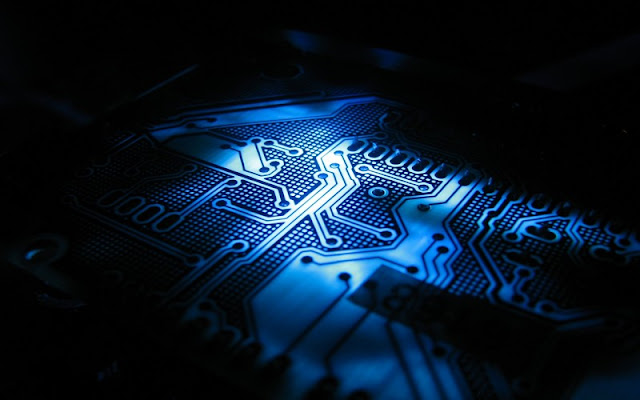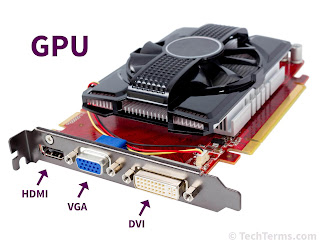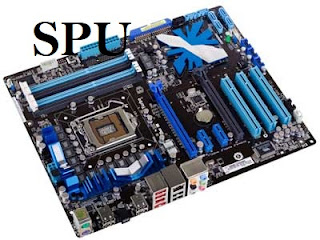# Evaluation of 8,16,32 and 64 bit computing

## How our processing units become more powerful.

So, I tell you one thing that the real magic comes with our processing units.Processing Unit of a computer system

Our processing units become more powerful and evaluate because they composed more once and not at a single time and giving us more range.

### So let's understand how our computer become more powerful.8-bit computer system processing

#### (i) Unsigned 8 Bit System:-

What we want to do let's find out possible combinations number of 1 and 0 there is.
So we work with the 8-bit system and the range of the 8-bit system is 256(including zero) possibilities.
0 to 255
in 8 bit system, there are GPU, SPU, and CPU.

you learn about the GPU, SPU, and CPU in my earlier chapter.How the GPU works in the different operating system?

GPU:-
When we sand 256 possible binary numbers to the GPU with
0  = Black color
255 = White color
and any possible number between two points produced a color like green, red.

So, we have 256 possible colors.How the SPU works?
SPU:-
So we have 256 possible binary combinations and 256 possible sound waves.
but the 256 sound waves is not sufficient
for hear.
So our sounds get a little bit tinny.working of CPU

CPU:-
So we have only 255 maximum combinations to produce output and maximum 255 number we get. and go only at  255 levels.

So as well as in 16, 32, and 64 bit systems.
the output depends on an only range of bit system.
Ex:-
As an example, the range of the 16-bit system is 0 to 65,535. so we get 65,536 possible bases two numbers.
So, in GPU we get 65,535 colors, sound waves and mathematical levels in the 16-bit system.

#### EVALUATION OF BIT SYSTEM

So, I gonna give you a  task find the range of 32 and 64 bit systems and comment on the section given below.

for any question please comment on the section given below.